If there is an Integersuch that(1)

thenis said to be a quadratic residue (mod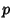). If not,is said to be a quadratic nonresidue (mod). For example,, so 6 is a quadratic residue (mod 10). The entire set of quadratic residues (mod 10) are given by 1, 4, 5, 6, and 9, since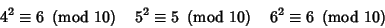making the numbers 2, 3, 7, and 8 the quadratic nonresidues (mod 10).

A list of quadratic residues for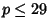is given below (Sloane's A046071), with those numbers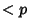not in the list being quadratic nonresidues of.Quadratic Residues 1 (none) 2 1 3 1 4 1 5 1, 4 6 1, 3, 4 7 1, 2, 4 8 1, 4 9 1, 4, 7 10 1, 4, 5, 6, 9 11 1, 3, 4, 5, 9 12 1, 4, 9 13 1, 3, 4, 9, 10, 12 14 1, 2, 4, 7, 8, 9, 11 15 1, 4, 6, 9, 10 16 1, 4, 9 17 1, 2, 4, 8, 9, 13, 15, 16 18 1, 4, 7, 9, 10, 13, 16 19 1, 4, 5, 6, 7, 9, 11, 16, 17 20 1, 4, 5, 9, 16

Given an Odd Primeand an Integer, then the Legendre Symbol is given by(2)

If(3)

then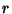is a quadratic residue (+) or nonresidue (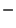). This can be seen since ifis a quadratic residue of, then there exists a squaresuch that, so(4)

andis congruent to 1 (mod) by Fermat's Little Theorem.is given by(5)

More generally, letbe a quadratic residue modulo an Odd Prime. Choose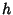such that the Legendre Symbol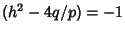. Then defining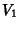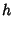(6)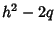(7)(8)

gives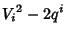(9)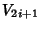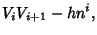(10)

and a solution to the quadratic Congruence is(11)

The following table gives the Primes which have a given number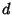as a quadratic residue.Primes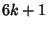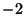23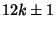56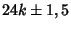Finding the Continued Fraction of a Square Rootand using the relationship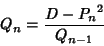(12)

for theth Convergentgives(13)

Therefore,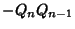is a quadratic residue of. But since,is a quadratic residue, as must be. But sinceis a quadratic residue, so is, and we see that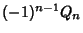are all quadratic residues of. This method is not guaranteed to produce all quadratic residues, but can often produce several small ones in the case of large, enablingto be factored.

The number of Squaresinis related to the numberof quadratic residues inby(14)

for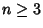(Stangl 1996). Bothand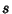are Multiplicative Functions.

References

Burton, D. M. Elementary Number Theory, 4th ed. New York: McGraw-Hill, p. 201, 1997.

Courant, R. and Robbins, H. Quadratic Residues.'' §2.3 in Supplement to Ch. 1 in What is Mathematics?: An Elementary Approach to Ideas and Methods, 2nd ed. Oxford, England: Oxford University Press, pp. 38-40, 1996.

Guy, R. K. Quadratic Residues. Schur's Conjecture'' and Patterns of Quadratic Residues.'' §F5 and F6 in Unsolved Problems in Number Theory, 2nd ed. New York: Springer-Verlag, pp. 244-248, 1994.

Niven, I. and Zuckerman, H. An Introduction to the Theory of Numbers, 4th ed. New York: Wiley, p. 84, 1980.

Rosen, K. H. Ch. 9 in Elementary Number Theory and Its Applications, 3rd ed. Reading, MA: Addison-Wesley, 1993.

Shanks, D. Solved and Unsolved Problems in Number Theory, 4th ed. New York: Chelsea, pp. 63-66, 1993.

Sloane, N. J. A. Sequence A046071 in The On-Line Version of the Encyclopedia of Integer Sequences.'' http://www.research.att.com/~njas/sequences/eisonline.html.

Stangl, W. D. Counting Squares in.'' Math. Mag. 69, 285-289, 1996.

Wagon, S. Quadratic Residues.'' §9.2 in Mathematica in Action. New York: W. H. Freeman, pp. 292-296, 1991.